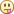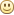Co-founder @ SMERGERS and WealthBox.in

## Facebook Hacker Cup Qualifiers 201330-Jan-2013

Hacker cup has got a lot better than what it used to be a few years back. I did like the problem set. It was good for a qualifying round. There is one significant rule which bothers me to some extentWe are not allowed to make multiple submissions after the 6 minute timeline even if the contest is still on. This is different from every other programming competition I know of.

1. TopCoder - We can change our solution even after submission, it attracts a penalty, but its better than submitting a wrong answer.
2. CodeChef - We are notified about the correctness of the solution right then and we can always correct it. Penalty is the number of attempts.
3. CodeForces - Here, we can lock a solution if we are confident. Otherwise, we can continue to resubmit it any number of times.

I made a mistake in the second problem but I could not correct it due to the single submission rule. One of my friend had swapped the source and output files by mistake and there was no error thrown. Hope these things are taken care of in the subsequent years. Here are my solutions for the problems.

#### Beautiful Strings

When John was a little kid he didn't have much to do. There was no internet, no Facebook, and no programs to hack on. So he did the only thing he could... he evaluated the beauty of strings in a quest to discover the most beautiful string in the world.

Given a string s, little Johnny defined the beauty of the string as the sum of the beauty of the letters in it.

The beauty of each letter is an integer between 1 and 26, inclusive, and no two letters have the same beauty. Johnny doesn't care about whether letters are uppercase or lowercase, so that doesn't affect the beauty of a letter. (Uppercase 'F' is exactly as beautiful as lowercase 'f', for example.)

You're a student writing a report on the youth of this famous hacker. You found the string that Johnny considered most beautiful. What is the maximum possible beauty of this string?

Input
The input file consists of a single integer m followed by m lines.
Output
Your output should consist of, for each test case, a line containing the string "Case #x: y" where x is the case number (with 1 being the first case in the input file, 2 being the second, etc.) and y is the maximum beauty for that test case.
Constraints
5 ≤ m ≤ 50
2 ≤ length of s ≤ 500

#### Balanced Smileys

Your friend John uses a lot of emoticons when you talk to him on Messenger. In addition to being a person who likes to express himself through emoticons, he hates unbalanced parenthesis so much that it makes him go :(

Sometimes he puts emoticons within parentheses, and you find it hard to tell if a parenthesis really is a parenthesis or part of an emoticon.

A message has balanced parentheses if it consists of one of the following:

- An empty string ""
- One or more of the following characters: 'a' to 'z', ' ' (a space) or ':' (a colon)
- An open parenthesis '(', followed by a message with balanced parentheses, followed by a close parenthesis ')'.
- A message with balanced parentheses followed by another message with balanced parentheses.
- A smiley face "" or a frowny face ":("
Write a program that determines if there is a way to interpret his message while leaving the parentheses balanced.

Input
The first line of the input contains a number T (1 ≤ T ≤ 50), the number of test cases.
The following T lines each contain a message of length s that you got from John.

Output
For each of the test cases numbered in order from 1 to T, output "Case #i: " followed by a string stating whether or not it is possible that the message had balanced parentheses. If it is, the string should be "YES", else it should be "NO" (all quotes for clarity only)

Constraints
1 ≤ length of s ≤ 100

#### Find the Min

After sending smileys, John decided to play with arrays. Did you know that hackers enjoy playing with arrays? John has a zero-based index array, m, which contains n non-negative integers. However, only the first k values of the array are known to him, and he wants to figure out the rest.

John knows the following: for each index i, where k <= i < n, m[i] is the minimum non-negative integer which is *not* contained in the previous *k* values of m.

For example, if k = 3, n = 4 and the known values of m are [2, 3, 0], he can figure out that m = 1.

John is very busy making the world more open and connected, as such, he doesn't have time to figure out the rest of the array. It is your task to help him.

Given the first k values of m, calculate the nth value of this array. (i.e. m[n - 1]).

Because the values of n and k can be very large, we use a pseudo-random number generator to calculate the first k values of m. Given non-negative integers a, b, c and positive integer r, the known values of m can be calculated as follows:

m = a
m[i] = (b * m[i - 1] + c) % r, 0 < i < k

Input
The first line contains an integer T (T <= 20), the number of test cases.
This is followed by T test cases, consisting of 2 lines each.
The first line of each test case contains 2 space separated integers, n, k (1 <= k <= 10^5, k < n <= 10^9).
The second line of each test case contains 4 space separated integers a, b, c, r (0 <= a, b, c <= 10^9, 1 <= r <= 10^9).
Output
For each test case, output a single line containing the case number and the nth element of m.